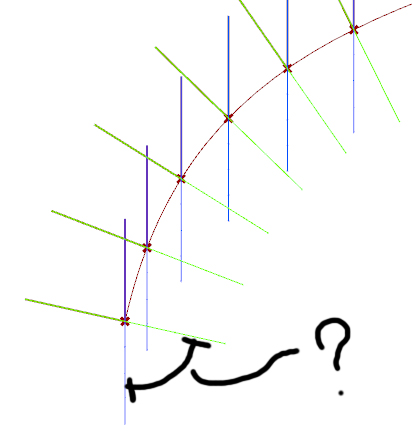# Obtain a list of rotation values of multiple geometry from a reference plane

Hi everybody,
as I wrote in the title, I need to obtain the rotation values of some plans that I constructud in grasshopper. I need to connect this values in Hummigbird, and bring the geometry that are oriented in the same direction of the plane into revit as a family.

I try to explain in a better way:

I have a curve that I divided with the component divide distance and than I created a plane for each of this point with the component Perpendicular Frame. In this way i have differents plans that correspond with the points.

I know that the plane YZ is the reference plane where the rotation value is 0.

How can obtain a list of rotation values in degree between the reference plane and the plane that is rotated?

I tried with the orient direction component, but it doesn’t have an output where extract this value.In blue are the reference plans with null rotation value and in green the plans that I need to obtain the rotation value.

Can someone help me?
Thanks in advanceRotation values.gh (10.8 KB)

Check if this does what you want:

Q_Rotation values.gh (17.6 KB)

// Rolf

1 Like

yes exactlywas really easy at the end! thank you so much!